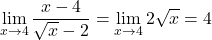## lim x->4 [( x-4 / x^(1/2)-2 ) ] Question related to limits . It’s lim x arrow to 4 . Try to answer this hardcor

Question

lim x->4 [( x-4 / x^(1/2)-2 ) ]

Question related to limits .
It’s lim x arrow to 4 .
Try to answer this hardcore question.

in progress 0
6 months 2021-08-30T10:22:21+00:00 1 Answers 3 views 0

## Answers ( )

1.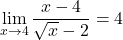Step-by-step explanation: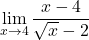A straightforward substitution of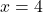will give us an indeterminate solution. So we use L’Hopital’s rule which states that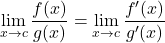where f'(x) and g'(x) are the derivatives of f(x) and g(x), respectively. We can see that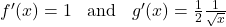Therefore,